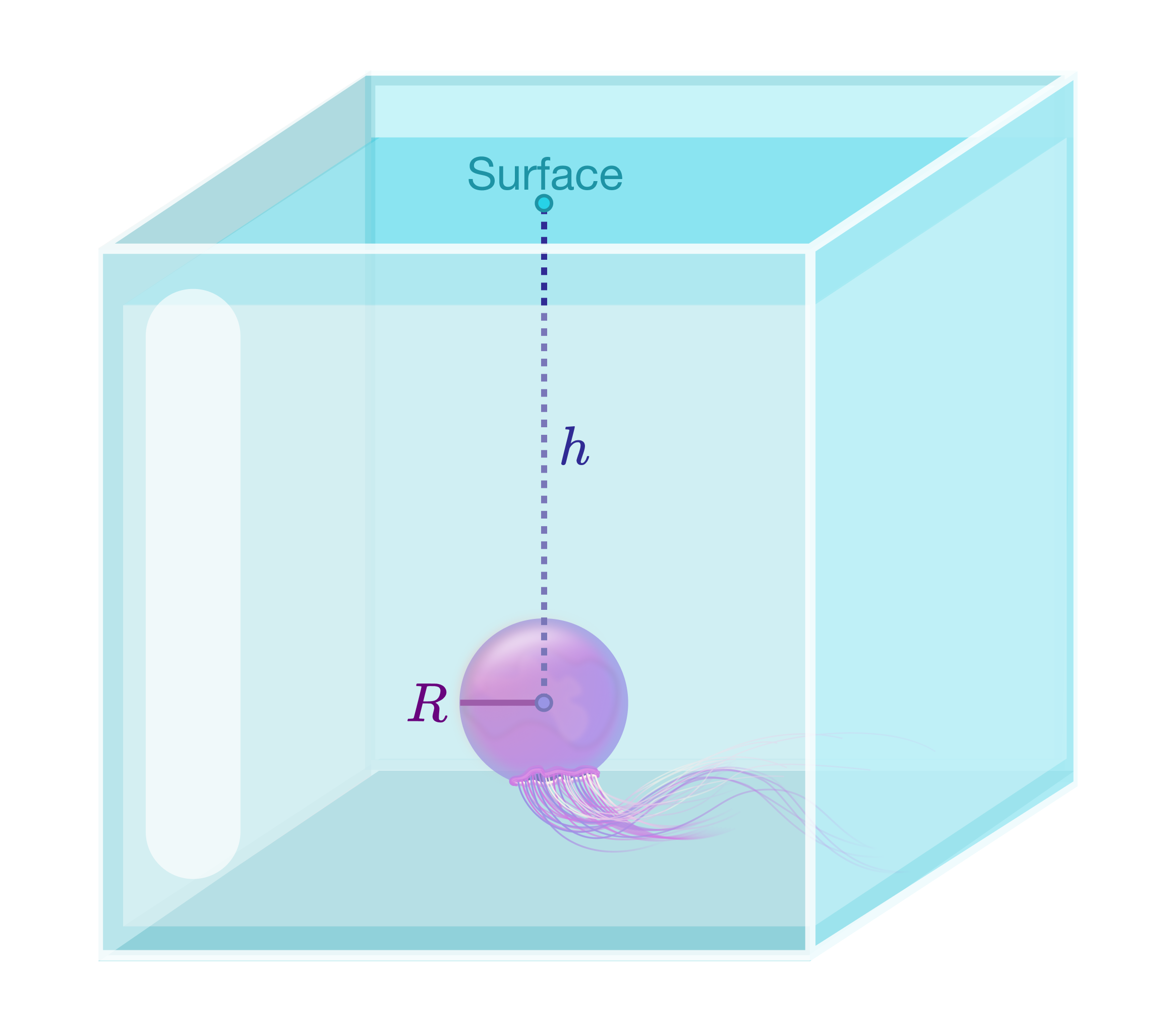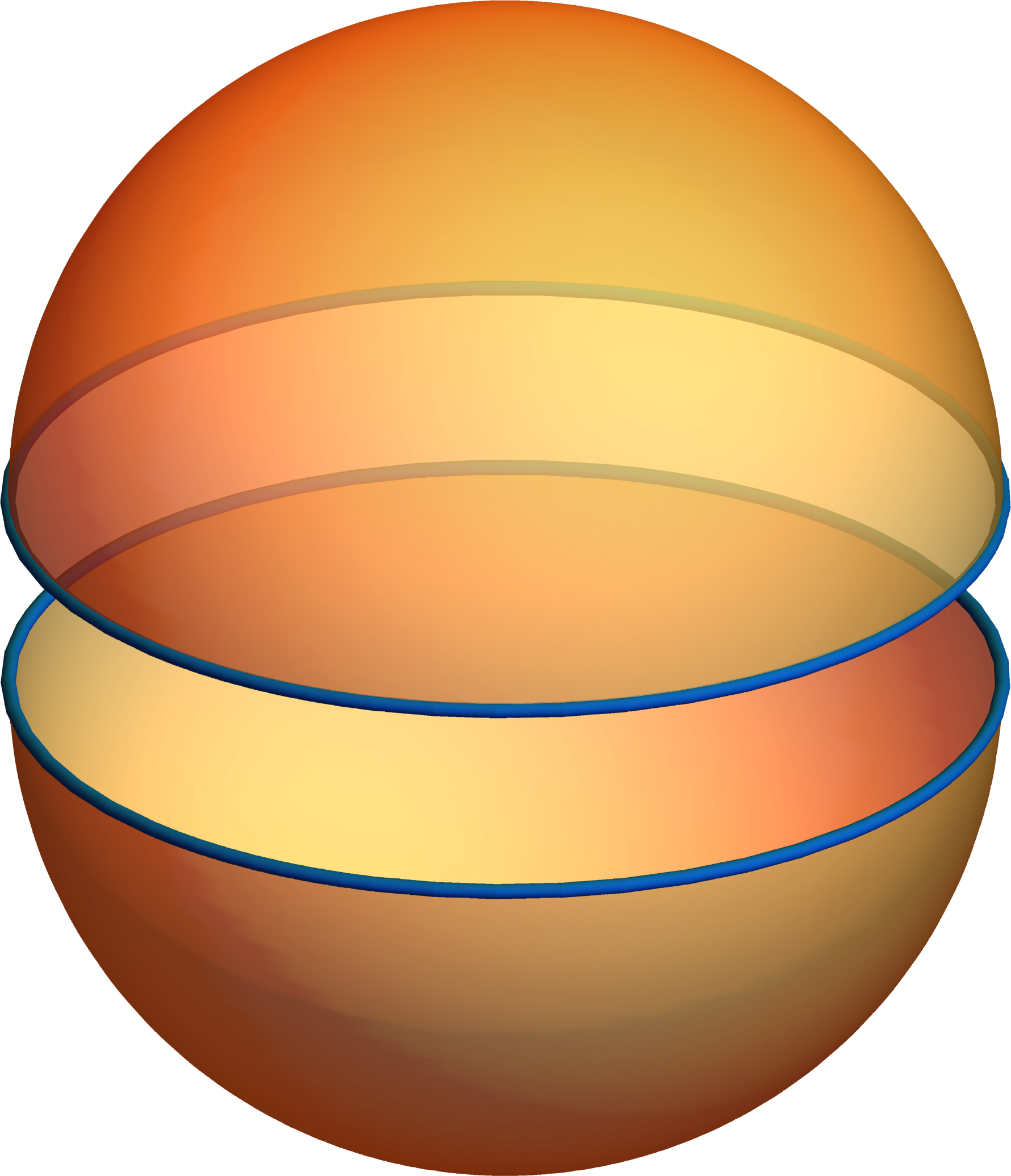### Vector Calculus

In the last quiz, we computed the hydrostatic force on the walls $S$ of two different aquariums using a new kind of integral:$\vec{F}_{\text{tot}} = \iint_{S} p \ \hat{n}\, dA.$ Since the outward unit normal $\hat{n}$ changes from point to point on $S,$ it is a vector field and so is $p ~ \hat{n}.$ The total force is therefore a vector field integral.

This quiz continues our work with hydrostatic pressure. Using the surface integral above, we'll uncover one of the greatest results of vector calculus: the Divergence Theorem.

# Introducing the Divergence TheoremTanks For All The Fish is designing a new home for Gilly the Jellyfish. Everyday she inflates into a full, round sphere of radius $R$ and sits at the very bottom of her tank.

To make sure she's comfortable, they need to calculate the total hydrostatic force $\vec{F}_{\text{tot}}$ Gilly feels due to the water inside her.

Place a coordinate system at Gilly's center (which is $h$ units below the water's surface$)$ with $z$ pointing vertically up. Then $\vec{F}_{\text{tot}} = \iint_{S} p \ \hat{n}\, dA = p_{0} \iint_{S} \left[ 1- \frac{z}{h} \right]\hat{n}\, dA.$ Here, $p_{0}$ is the pressure she feels on her equator, and $\hat{n}$ points perpendicularly outward.

What is the correct expression for $\hat{n}(x,y,z)$ as a vector field on Gilly's surface $S ?$

# Introducing the Divergence Theorem

After bringing constants outside the integral, we have $\vec{F}_{\text{tot}} = \iint_{S} p ~ \hat{n}\, d A = \frac{p_{0}}{R} \iint_{S} \left[ 1- \frac{z}{h} \right] \langle x, y, z \rangle\, d A.$ We can use symmetry to get us to the right answer quickly.

For example, recall that $\int_{-1}^{1} x^3 \, dx = 0$ since there is equal area above and below the curve $y = x^{3}$ due to the odd symmetry $f(-x) = - f(x)$ of $f(x) =x^3.$

Using symmetry alone, what component(s) of $\vec{F}_{\text{tot}}$ have to be 0?

# Introducing the Divergence Theorem

Select one or moreThrough symmetry the total hydrostatic force reduces to $\vec{F}_{\text{tot}} = \frac{p_{0}}{R} \iint_{S} \left[ 1- \frac{z}{h} \right] z \, d A\, \hat{k}.$ Now we're in a bind since Gilly is a full sphere $S,$ which cannot be represented as the graph of a single function like our hemispherical aquarium tank.

We could split poor Gilly along her equator; since both hemispheres are graphs, we can integrate as before and add the two results.

A better option (as Gilly would agree) represents $S$ as a single piece. Any point in $\mathbb{R}^3$ can be described in terms of spherical coordinates $x = \rho \sin(\phi) \cos(\theta),\quad y = \rho \sin(\phi) \sin(\theta),\quad z = \rho \cos( \phi).$ If we choose $\rho = R,$ we get every point of $S$ in terms of the two angles $\theta$ and $\phi.$ This is just what we need! The visualization below shows how varying the two spherical angles in $\vec{x}(\theta,\phi) = \big\langle R \sin(\phi) \cos(\theta), R \sin(\phi) \sin(\theta), R \cos( \phi) \big\rangle$ traces out every point on $S,$ just as $\vec{p} + t \vec{v}$ traces out every point on $L.$

# Introducing the Divergence Theorem

Challenge Problem:

To integrate and find the total hydrostatic force using the sphere representation $\vec{x}(\theta,\phi) = \big\langle R \cos(\theta) \sin(\phi), R \sin(\theta) \sin(\phi) , R \cos(\phi) \big\rangle,\ \ \theta \in [0,2\pi),\ \ \phi \in [0,\pi],$ we need to first work out the $d A$ in $\vec{F}_{\text{tot}} = \frac{p_{0}}{R} \iint_{S} \left[ 1- \frac{z}{h} \right] z \, d A \, \hat{k}.$ From the aquarium quiz, we know that in terms of Cartesian coordinates $d A = \frac{dx\, dy}{\sqrt{1- \frac{x^2+y^2}{R^2}}}.$

In terms of $\theta$ and $\phi,$ however, $dA = \text{\_\_\_\_\_\_\_\_\_\_\_\_\_}\, d \theta\, d \phi.$

Fill in the blank.

Hint: Switching from Cartesian coordinates $x$ and $y$ to spherical coordinates $\theta$ and $\phi$ requires the use of a Jacobian determinant: $dx\, dy = \left | \det \begin{pmatrix} \frac{\partial x}{\partial \theta} & \frac{\partial x}{\partial \phi} \\ \frac{\partial y}{\partial \theta} & \frac{\partial y}{\partial \phi} \end{pmatrix} \right |\, d\theta\, d \phi.$

# Introducing the Divergence Theorem

In terms of the spherical angles, we have $\vec{F}_{\text{tot}} = \frac{p_{_0}}{R} \int\limits_{\theta=0}^{\theta = 2\pi}\int\limits_{\phi=0}^{\phi = \pi} \Bigg [ 1- \frac{\overbrace{R\cos(\phi)}^{z}}{h} \Bigg ] \overbrace{R\cos(\phi)}^{z} ~ \overbrace{R^2\sin(\phi)\, d \phi\, d \theta}^{dA} \, \hat{k}.$ Use the substitution $u = \cos(\phi)$ to evaluate this integral.

# Introducing the Divergence Theorem

We notice something very interesting about our result: $\vec{F}_{\text{tot}} = \underbrace{\frac{4 \pi R^3}{3}}_{\text{Gilly's volume}} \overbrace{\left( -\frac{p_{_0}}{h} \right)}^{\text{constant}} \hat{k}.$ Since the volume of a ball $B$ of radius $R$ can also be written as the triple integral $\frac{4 \pi R^3}{3} = \iiint\limits_{B} 1\, dx\, dy\, dz,$ the total hydrostatic force can be written as a triple integral involving the pressure $p.$

What is this triple integral given that $p = p_{_0} \left[ 1- \frac{z}{h} \right]?$

# Introducing the Divergence Theorem

By calculating the total hydrostatic force on Gilly's inside, we're led to a rather striking result: $\vec{F}_{\text{tot}} = \iiint\limits_{B} \nabla p\, d\vec{x} = \iint\limits_{S} p\, \hat{n}\, dA.$ It turns out this formula is true if we replace $p$ with a general function $f,$ and think of $B$ as a general region in $\mathbb{R}^3$ with an outward-pointing unit normal $\hat{n}$ along the boundary surface $\partial B = S.$

In fact, this is just one possible form of the Divergence Theorem! In the final few problems, we'll use the formula $\iiint\limits_{B} \nabla f\, d\vec{x} = \iint\limits_{\partial B} f\, \hat{n}\, dA$ to make contact with the form of the Divergence Theorem we saw in the nutshell quiz.

# Introducing the Divergence Theorem

Challenge Problem:

Suppose we have a 3D vector field $\vec{V}(\vec{x}).$ We can express $\iint\limits_{\partial B} \vec{V} \cdot \hat{n}\, dA$ as a triple integral using the identity $\iiint\limits_{B} \nabla f\, d\vec{x} = \iint\limits_{\partial B} f\, \hat{n}\, dA.$ What is the integrand? In other words, fill in the blank: $\iint\limits_{\partial B} \vec{V} \cdot \hat{n}\, dA = \iiint\limits_{B} \text{\_\_\_\_\_\_\_\_\_\_\_\_\_\_\_\_}\, d\vec{x}.$

# Introducing the Divergence Theorem

Gilly rests easier in water with a lot of oxygen dissolved in it, so we set up a jet of oxygen-rich water along the bottom of the tank. The jet current points along the $x$-direction and has constant magnitude:

Let's write the jet as $\vec{J} = j_{0} \hat{i},$ where $j_{0}$ has units of mass (of oxygen) per unit time per unit area. The total rate at which oxygen enters/leaves through Gilly's surface $S$ per unit time is $\iint\limits_{S} \vec{J} \cdot \hat{n}\, d A.$ Here $S$ is the sphere of radius $R$ centered at the origin. Compute this integral.

Hint: You could evaluate the integral directly or you could use the Divergence Theorem: $\iint\limits_{S} \vec{J} \cdot \hat{n}\, d A = \iiint\limits_{B} \nabla \cdot \vec{J}\, d \vec{x} = \iiint\limits_{B} \left[ \frac{\partial J_{x}}{\partial x} + \frac{\partial J_{y}}{\partial y} + \frac{\partial J_{z}}{\partial z} \right] d \vec{x}.$ $B$ is the inside of the sphere $S.$ As a bonus, try both ways and see that you get the same result.

# Introducing the Divergence Theorem

We hope you enjoyed this brief intro to the basic ideas of vector calculus.

Our next series of quizzes, Vector-valued Functions, picks up the story of vectors and motion.

Later quizzes develop the divergence and curl vector derivatives as well as their surface integral and line integral counterparts.

The finale brings everything in the course together to solve important real-world problems.

# Introducing the Divergence Theorem

×